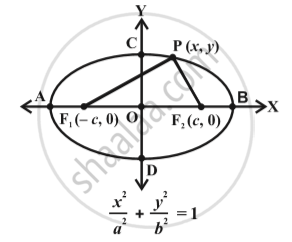# Ellipse - Standard Equations of an Ellipse

#### notes

The  equation of  an ellipse is simplest if the centre of the ellipse is at the origin and the foci areon the x-axis or y-axis.  The two such possible orientations are shown in above Fig.
We will derive the equation for the ellipse shown above in Fig  with foci on the x-axis.
Let F_1 and F_2 be the foci and O be the midpoint of the line segment
F_1F_2. Let O be the origin and the line from O through F_2 be the positive x-axis and that through F_1as the negative x-axis. Let, the line through O perpendicular to the x-axis be the y-axis. Let the coordinates of F_1 be
(– c, 0) and F_2 be (c, 0).
Let P(x, y) be any point on the ellipse such that the sum of the distances from P to the two foci  be 2a so given
PF_1 + PF_2 = 2a.           ... (1)
Using the distance formula,
sqrt((x + c)^2 + y^2) + sqrt((x - c )^2 + y^2)  2a

i.e., sqrt ((x + c)^2 + y^2 ) = 2a - sqrt((x - c )^2 + y^2)

Squaring both sides, we get

(x + c)^2 + y^2 = 4a^2 – 4a sqrt((x-c)^2 + y^2) + (x - c)^2 + y^2

which on simplification gives

sqrt((x - c)^2 + y^2) = a-(c/a) x
Squaring again and simplifying, we get
x^2/a^2 + y^2/(a^2 - c^2) = 1

i.e., x^2/a^2 + y^2/b^2 = 1
Hence any point on the ellipse satisfies
x^2/a^2 + y^2/b^2 = 1

The standard equation of the ellipses for the above Fig.

1. Ellipse is symmetric with respect to both the coordinate axes since if (x, y) is a point on the ellipse, then (– x, y), (x, –y) and (– x, –y) are also points on the ellipse.

2. The foci  always lie on the major axis.  The major axis can be determined by finding the intercepts on the axes of symmetry.  That is, major axis is along the x-axis if the coefficient of x^2 has the larger denominator and it is along the y-axis if the coefficient of  y^2 has the larger denominator.

If you would like to contribute notes or other learning material, please submit them using the button below.

### Shaalaa.com

Standard equations of an ellips [00:03:01]
S
0%